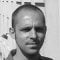Get access to premium HV/MV/LV technical articles, electrical engineering guides and research studies. Check new membership plans!

# Power flow in MV radial network and calculation of voltage drop and loss

Home / Technical Articles / Power flow in MV radial network and calculation of voltage drop and loss

## Power flow in radial network

In three phase system the load for each phase should be the same. We call symmetrical load when the load of each phase is equal.

In symmetrical circuit each phase has same configuration, so in drawing we can represent only one phase and omit 2 other phases. The diagram that shows only one line is called single line diagram.

The load of different types has different representations. In the power system the best presentation is power load S = (P, Q).

The point to where the load is connected is called node. Ordinary this is may be a feeder, a derivation of MV network, a transformer post. The tension at this point we call node voltage.

### Electrical scheme, single diagram

Suppose the loads are symmetrical. So for phase A the scheme is the same as for phase B or phase C. To show one phase is sufficient.

At the beginning node of a branch:

• Node voltage by U1,
• Active power flow from the beginning by P’ and
• Reactive power flow by Q’

At the end node of a branch:

• Node voltage by U2,
• Active power flow to the end node by P” and
• Reactive power flow by Q”

Voltage drop has 2 components, a longitudinal and a transversal component.

• DU2 = (P” r + Q”x)/U2 (longitudinal)
• dU2 = (P” x – Q” r)/U2 (transversal)
• DU1 = (P’ r + Q’ x)/U1
• dU1 = (P’ x – Q’ x)/U1

In general when the power factor is about 0.8 the transversal component of voltage drop is small compare to the longitudinal one. dU2 can be drop out in calculation.

Node voltage:
U1 = √((U2 + DU2)2 + dU22)
U2 =√((U1-DU1)2 + dU12)

In normal case, when power factor is greater than 0.8:
U1 ≈ U2 + DU2
U2 ≈ U1 – DU1

Voltage loss:
DU = U1 – U2

Power loss:
DP = ( P”2 + Q”2) r/U22 DQ = (P”2 + Q”2) x/U22
DP = ( P’2 + Q’2) r/U12 DQ = (P’2 + Q’2) x/U12

Radial network is a network whose configuration does not contain any loop (tree like configuration).

#### Principles of calculation (an example)

 Beginning Ending Branch Comment A B AB B C BC C D CD D E DE E is last node B F BF F is last node D G DG G H GH H is last node

To find out the path from the first node to last node:

1. Write down branch of last node, ex: DE
2. Get the previous node to the node D: C so we have CDE
3. Repeat this process until the first node
BCDE
ABCDE

For the last node F: ABF
For the last node H: ABCDGH

1. Find the path to each last node
2. Compute the power flow for each branch
3. From the first to the last node compute all node voltage, voltage drop in each branch and power loss.

#### Example of radial network calculation

1. The path is {0, 1, 2}

2. From node 2 the power flows are:

P12 = P2 + 0 = P2 = 1 MW
Q12 = Q2 + 0 = Q2 = 0.6 MVar

From node 1 the power flows are:

P01 = P1 + P12 = 0.8 + 1 = 1.8 MW
Q01 = Q1 + Q12 = 0.3 + 0.6 = 0.9 MVar

3. Compute voltage drop and power loss:

From node 0 to 1 (branch { 0, 1})

DU1(01) = (P01.r01 + Q01.x01)/U0 = (1.8 x 1+0.9 x 1)/22 = 0.123 kV
U1 = U0 – DU1 = 21.877 kV

From node 1 to node 2 (branch {1, 2})

DU1(12) = (P12.r12+Q12.x12)/U1 = (1 x 1.5 + 0.6 x 1)/21.877 = 0.096 kV
U2 = U1 – DU1(12) = 21.877 – 0.096 = 21.781 kV

Total voltage drop = 22 – 21.781 = 0.219 kv that means 1%.

Reference // Handbook for installation of medium voltage lines by Mr. Ky Chanthan, Mr. Theng Marith

Get access to premium HV/MV/LV technical articles, electrical engineering guides, research studies and much more! It helps you to shape up your technical skills in your everyday life as an electrical engineer.### Edvard Csanyi

Electrical engineer, programmer and founder of EEP. Highly specialized for design of LV/MV switchgears and LV high power busbar trunking (<6300A) in power substations, commercial buildings and industry facilities. Professional in AutoCAD programming.

1.Shandukani Vhulondo
Jul 02, 2017

Thank You very much for sharing such valuable information

•Edvard
Jul 03, 2017

You’re welcome Shandukani.

2.José Jamal
Jul 01, 2017

Thanks for sharing such a wonderful issue. It is likely to be forgoten with time.

•Edvard
Jul 03, 2017

3.Alfred Gjylameti
Nov 02, 2016

Hi Mr. Edvart

I need a design program for 20kV overhead transmission lines. May you help me?

Best Regards
Alfred

•vujini sudhakar
Dec 07, 2017

i can give the design of 20 kv line , provided you need to give info about location and load Etc.

### Subscribe to Weekly Digest

Get email alert whenever we publish new electrical guides and articles.